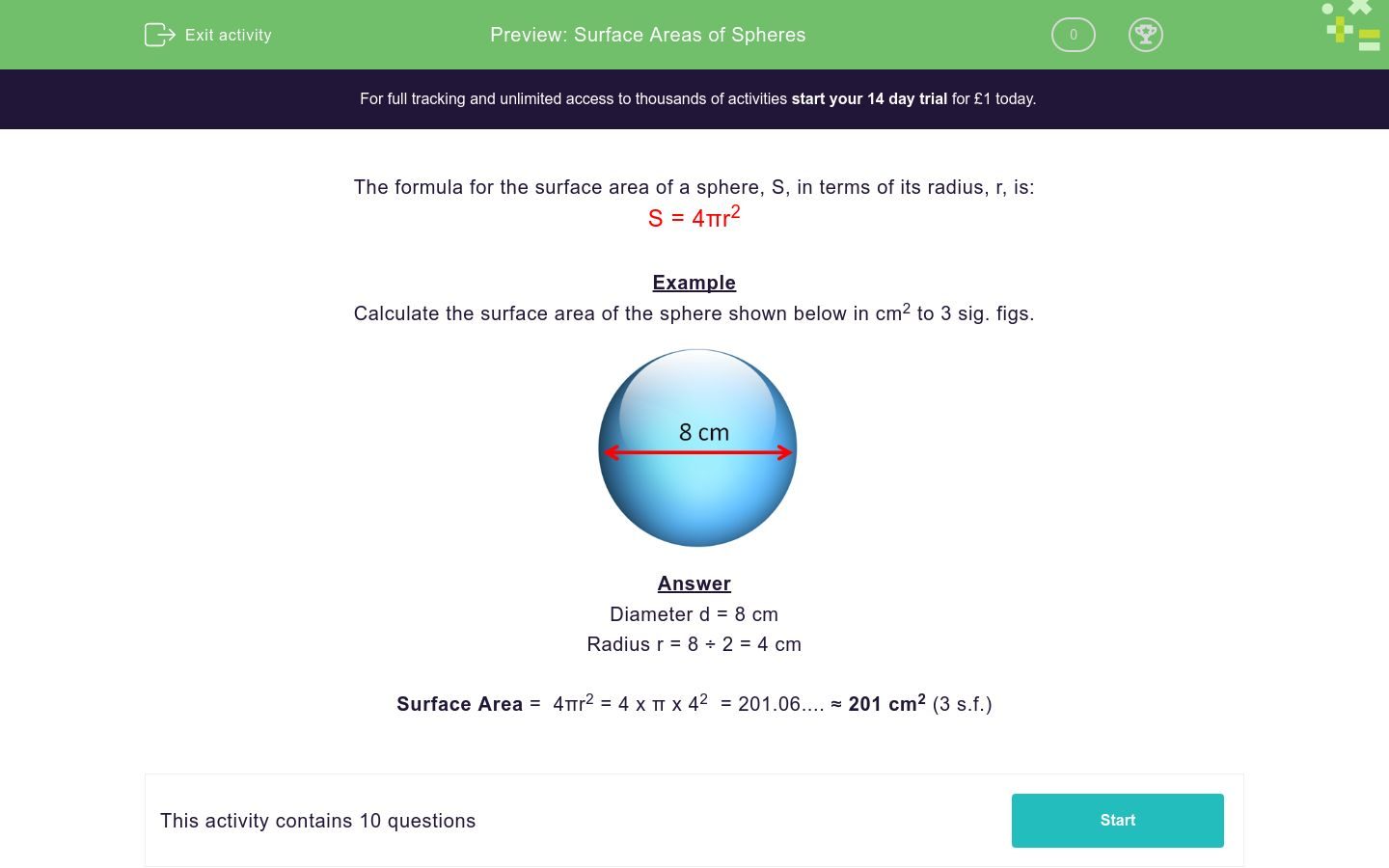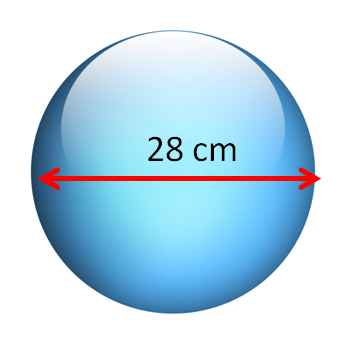# Surface Areas of Spheres

In this worksheet, students calculate the surface area of spheres, given their radii or diameters.Key stage:  KS 3

Curriculum topic:   Geometry and Measures

Curriculum subtopic:   Use 3D Shape Knowledge to Solve Problems

Difficulty level:### QUESTION 1 of 10

The formula for the surface area of a sphere, S, in terms of its radius, r, is:

S = 4πr2

Example

Calculate the surface area of the sphere shown below in cm2 to 3 sig. figs.Diameter d = 8 cm

Radius r = 8 ÷ 2 = 4 cm

Surface Area =  4πr2 = 4 x π x 42  = 201.06.... ≈ 201 cm2 (3 s.f.)

Calculate the surface area of the sphere shown below in cm2 to 3 sig. figs.

(just write the number)Calculate the surface area of the sphere shown below in cm2 to 3 sig. figs.

(just write the number)Calculate the surface area of the sphere shown below in cm2 to 3 sig. figs.

(just write the number)Calculate the surface area of the sphere shown below in cm2 to 3 sig. figs.

(just write the number)Calculate the surface area of the sphere shown below in cm2 to 3 sig. figs.

(just write the number)Calculate the surface area of the sphere shown below in cm2 to 3 sig. figs.

(just write the number)Calculate the surface area of the sphere shown below in cm2 to 3 sig. figs.

(just write the number)Calculate the surface area of the sphere shown below in cm2 to 3 sig. figs.

(just write the number)Calculate the surface area of the sphere shown below in cm2 to 3 sig. figs.

(just write the number)Calculate the surface area of the sphere shown below in cm2 to 3 sig. figs.

(just write the number)• Question 1

Calculate the surface area of the sphere shown below in cm2 to 3 sig. figs.

(just write the number)113
EDDIE SAYS
Surface Area = 4 × π × 3²
• Question 2

Calculate the surface area of the sphere shown below in cm2 to 3 sig. figs.

(just write the number)804
EDDIE SAYS
Surface Area = 4 × π × 8²
• Question 3

Calculate the surface area of the sphere shown below in cm2 to 3 sig. figs.

(just write the number)2830
EDDIE SAYS
Surface Area = 4 × π × 15²
• Question 4

Calculate the surface area of the sphere shown below in cm2 to 3 sig. figs.

(just write the number)154
EDDIE SAYS
Surface Area = 4 × π × 3.5²
• Question 5

Calculate the surface area of the sphere shown below in cm2 to 3 sig. figs.

(just write the number)1020
EDDIE SAYS
Surface Area = 4 × π × 9²
• Question 6

Calculate the surface area of the sphere shown below in cm2 to 3 sig. figs.

(just write the number)2460
EDDIE SAYS
Surface Area = 4 × π × 14²
• Question 7

Calculate the surface area of the sphere shown below in cm2 to 3 sig. figs.

(just write the number)5030
EDDIE SAYS
Surface Area = 4 × π × 20²
• Question 8

Calculate the surface area of the sphere shown below in cm2 to 3 sig. figs.

(just write the number)452
EDDIE SAYS
Surface Area = 4 × π × 6²
• Question 9

Calculate the surface area of the sphere shown below in cm2 to 3 sig. figs.

(just write the number)908
EDDIE SAYS
Surface Area = 4 × π × 8.5²
• Question 10

Calculate the surface area of the sphere shown below in cm2 to 3 sig. figs.

(just write the number)7850
EDDIE SAYS
Surface Area = 4 × π × 25²
---- OR ----

Sign up for a £1 trial so you can track and measure your child's progress on this activity.

### What is EdPlace?

We're your National Curriculum aligned online education content provider helping each child succeed in English, maths and science from year 1 to GCSE. With an EdPlace account you’ll be able to track and measure progress, helping each child achieve their best. We build confidence and attainment by personalising each child’s learning at a level that suits them.

Get started